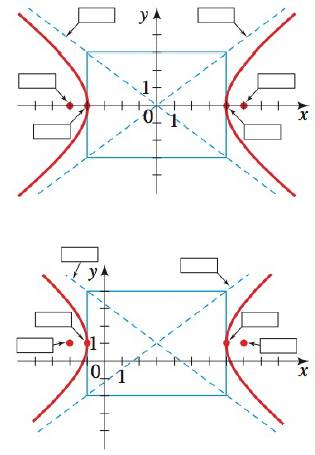Chapter 12.4, Problem 4E### Algebra and Trigonometry (MindTap ...

4th Edition
James Stewart + 2 others
ISBN: 9781305071742

#### Solutions

Chapter
Section### Algebra and Trigonometry (MindTap ...

4th Edition
James Stewart + 2 others
ISBN: 9781305071742
Textbook Problem

# CONCEPTS4. The graphs of x 2 4 2 − y 2 3 2 = 1 and ( x − 3 ) 2 4 2 − ( y − 1 ) 2 3 2 = 1 are given. Label the vertices, foci and asymptotes on each hyperbola.To determine

To find:

The vertices, foci and asymptotes of the hyperbola.

Explanation

Given:

The equations of hyperbola are,

x242y232=1(x3)242(y1)232=1

Approach:

The following equation is the basic equation for a hyperbola that is not centered at the origin.

(xh)2a2(yk)2b2=1

With the following properties,

Vertices V=(±a+h,k) ……(1)

Asymptotes (yk)=±ba(xh) ……(2)

Foci F=(±c+h,k) ……(3)

Here,

c2=a2+b2 ……(4)

Calculation:

Consider the equation,

x242y232=1

Convert the above equation into standard equation of hyperbola.

(x0)242(y0)232=1

Compare the above equation of the hyperbola with the standard equation a hyperbola.

a=4b=3h=0k=0

Calculate the vertices of the hyperbola.

Substitute 4 for a, 0 for h and 0 for k in equation (1).

V=(±4,0)

Calculate the asymptotes of the hyperbola.

Substitute 4 for a, 3 for b, 0 for h and 0 for k in equation (2).

y=±34x

Calculate the value of c.

Substitute 4 for a and 3 for b in equation (4).

c2=42+32c2=25c=±25=±5

Calculate the foci of the hyperbola.

Substitute 5 for c, 0 for h and 0 for k in equation (3).

F=(±5,0)

The vertices, foci and asymptotes of hyperbola x242y232=1 is shown in figure below,

Figure (1)

Consider the equation,

(x3)242(y1)232=1

Compare the above equation of the hyperbola with the standard equation a hyperbola

### Still sussing out bartleby?

Check out a sample textbook solution.

See a sample solution

#### The Solution to Your Study Problems

Bartleby provides explanations to thousands of textbook problems written by our experts, many with advanced degrees!

Get Started